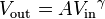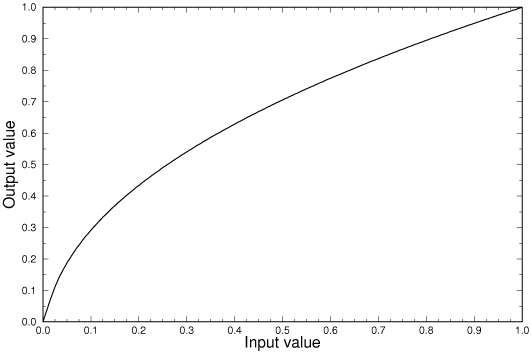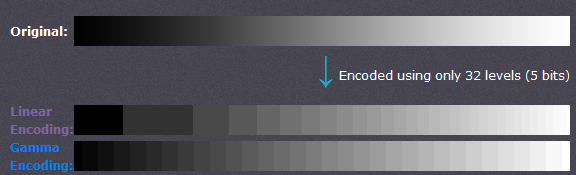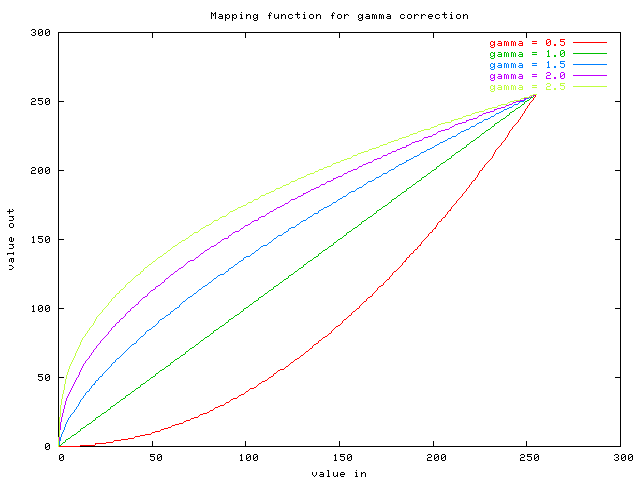# How to Use Gamma Correction for Image Processing with Opencv

In reality, we can always see some photos that have low brightnesses and low contrast. To make objects recognizable in pictures,  we need to process the photo with Illumination Compensation. There are many algorithms used for Illumination Compensation such as Histogram equalization, Color similarity measure, Gamma Correction and so on. In this tutorial, I will introduce Gamma Correction and show you how to use it with OpenCV.

## What is Gamma Correction

Gamma correction was originally designed to compensate for CRT monitors’ non-linear response to the input signal. CRTs were not able to amplify the input signal themselves and thus the output signal from the PC needed to be adjusted, giving rise to (as of today) standard gamma 2.2 correction and sRGB color space. Gamma Correction is the name of a nonlinear operation used to code and decode luminance or tristimulus values in video or still image systems. Here is the definition of Gamma Correction in Wikipedia:

“Gamma correction is, in the simplest cases, defined by the following power-law expression:where A is a constant and the input and output values are non-negative real values; in the common case of A = 1, inputs and outputs are typically in the range 0–1. A gamma value γ < 1 is sometimes called an encoding gamma, and the process of encoding with this compressive power-law nonlinearity is called gamma compression; conversely a gamma value γ > 1 is called a decoding gamma and the application of the expansive power-law nonlinearity is called gamma expansion.”Gamma encoded images store tones more efficiently. Since gamma encoding redistributes tonal levels closer to how our eyes perceive them, fewer bits are needed to describe a given tonal range. Otherwise, an excess of bits would be devoted to describing the brighter tones (where the camera is relatively more sensitive), and a shortage of bits would be left to describe the darker tones (where the camera is relatively less sensitive):If an image is under or over gamma corrected, this also affects the color balance. Over correction (in addition to making mid-tones too light) shifts colors towards neutral grey, while under correction (in addition to making mid-tones too dark) shifts colors towards the display primaries.We can see when the image has low brightnesses and low contrast, we can process it by Gamma Correction, and the value of gamma should be less than 1.Because the algorithm can expand low gray steps and compress the high gray steps when γ < 1.

## How to Use Gamma Correction with OpenCV

Gamma correction controls the overall brightness of an image. Images that are not corrected can look either bleached out or too dark. We can use this case:

``````R = pow(R, 1/Gamma)
G = pow(G, 1/Gamma)
B = pow(B, 1/Gamma)
``````

The algorithm can be implemented with the following code, which can process images that have one or three channels.

``````void GammaCorrection(Mat& src, Mat& dst, float fGamma)
{
unsigned char lut;
for (int i = 0; i < 256; i++)
{
lut[i] = saturate_cast<uchar>(pow((float)(i / 255.0), fGamma) * 255.0f);
}
dst = src.clone();

const int channels = dst.channels();
switch (channels)
{
case 1:
{
MatIterator_<uchar> it, end;
for (it = dst.begin<uchar>(), end = dst.end<uchar>(); it != end; it++)
*it = lut[(*it)];
break;
}
case 3:
{
MatIterator_<Vec3b> it, end;
for (it = dst.begin<Vec3b>(), end = dst.end<Vec3b>(); it != end; it++)
{
(*it) = lut[((*it))];
(*it) = lut[((*it))];
(*it) = lut[((*it))];
}
break;
}
}
}
``````

## How to Build and Run OpenCV Program on Ubuntu

Follow the official tutorial to install OpenCV 3.0.

``````#!/bin/bashd
#https://help.ubuntu.com/community/OpenCV
version="\$(wget -q -O - http://sourceforge.net/projects/opencvlibrary/files/opencv-unix | egrep -m1 -o '\"[0-9](\.[0-9]+)+' | cut -c2-)"
echo "Installing OpenCV" \$version
mkdir OpenCV
cd OpenCV
echo "Removing any pre-installed ffmpeg and x264"
sudo apt-get -qq remove ffmpeg x264 libx264-dev
echo "Installing Dependenices"
sudo apt-get -qq install libopencv-dev build-essential checkinstall cmake pkg-config yasm libjpeg-dev libjasper-dev libavcodec-dev libavformat-dev libswscale-dev libdc1394-22-dev libxine2-dev libgstreamer0.10-dev libgstreamer-plugins-base0.10-dev libv4l-dev python-dev python-numpy libtbb-dev libqt4-dev libgtk2.0-dev libfaac-dev libmp3lame-dev libopencore-amrnb-dev libopencore-amrwb-dev libtheora-dev libvorbis-dev libxvidcore-dev x264 v4l-utils ffmpeg cmake qt5-default checkinstall
echo "Installing OpenCV" \$version
unzip OpenCV-\$version.zip
cd opencv-\$version
mkdir build
cd build
cmake -D CMAKE_BUILD_TYPE=RELEASE -D CMAKE_INSTALL_PREFIX=/usr/local -D WITH_TBB=ON -D BUILD_NEW_PYTHON_SUPPORT=ON -D WITH_V4L=ON -D INSTALL_C_EXAMPLES=ON -D INSTALL_PYTHON_EXAMPLES=ON -D BUILD_EXAMPLES=ON -D WITH_QT=ON -D WITH_OPENGL=ON ..
make -j2
sudo checkinstall
sudo sh -c 'echo "/usr/local/lib" > /etc/ld.so.conf.d/opencv.conf'
sudo ldconfig
echo "OpenCV" \$version "ready to be used"
``````

Build the C++ program with g++:

``````g++ -ggdb `pkg-config --cflags opencv` -o `basename gamma.cpp .cpp` gamma.cpp `pkg-config --libs opencv`
``````

## Source Code

https://github.com/DynamsoftRD/opencv-programming/tree/master/gamma-correction